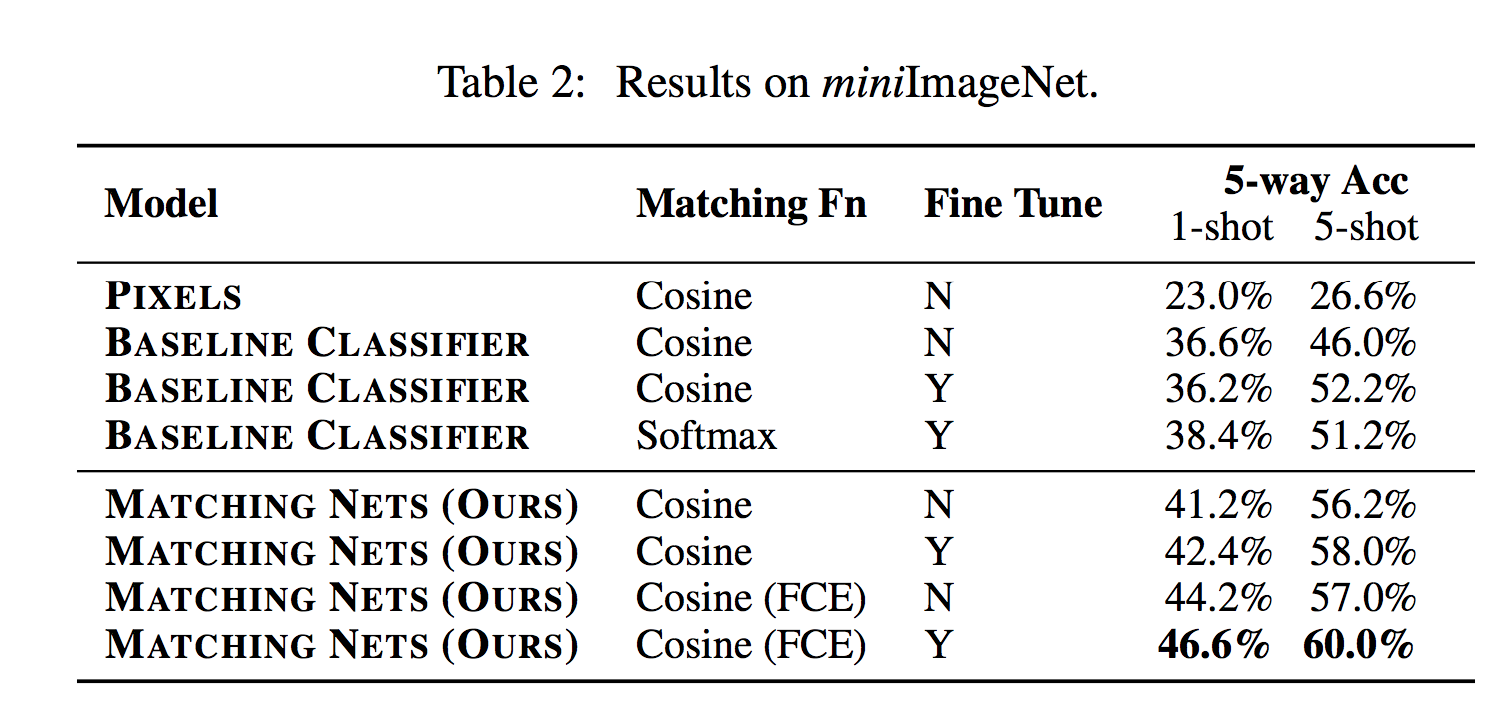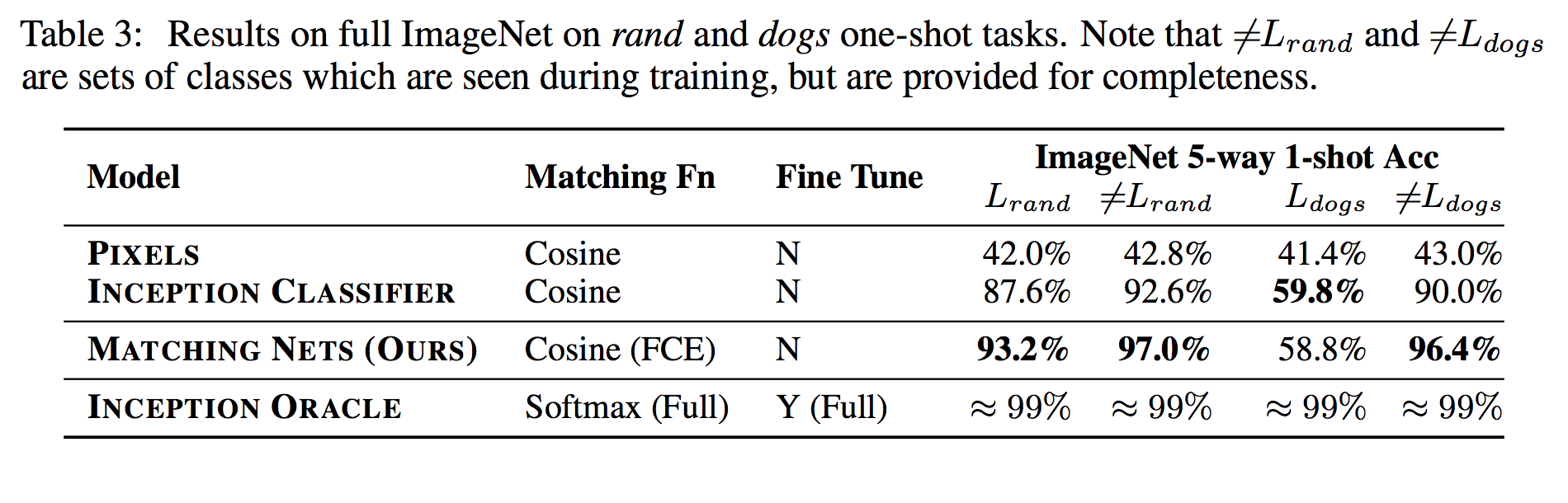### Description

This paper proposes a solution for small data sets which suffer from overfitting. The authors employ a metric learning which helps the network to rapidly learn from small data set. They applied this idea on a small set of Image Net and they improve an accuracy from 87.6% to 93.2%.

They use the advantages of one shot learning, but for prediction on the target, they investigate a metric to find how much an unseen sample is simmilar to set of seen samples in a episod and this similarity can be present as a weight on classes. This metric plays the same role as attention or a kernel function. The authors used a cosine distance on two vectors as a metric.

Suppose $$(x_{i},y_{i})$$ is a given (input, class) and $$\bar{x}$$ is a given input and predicted label $$\bar{y}$$ calculated as:

$\bar{y} = \sum_{i=1}^k a(\bar{x},x_i) y_{i}$

A small support set contains of $$k$$ label example with input $$x$$ and lable $$y$$, $$S= {(x_{i},y_{i})}_{i=1}^k$$, given a new example $$\bar{x}$$, we want to know the probability that it is an example of a given class $$P(\bar{y}\mid\bar{x},S)$$ where $$P$$ is parametrized by a neural network. Therefore $$S$$ maps to a classifier $$c_{s}(\bar{x})$$. Simply, suppose a task $$T$$ has a distribution over lable sets $$L$$.

First a set $$L$$ from task $$T$$ is sampled, Then from these sample labels, the support set $$S$$ and a batch $$B$$ are sampled. The Maching network has to minimize the prediction of lables in batch $$B$$ conditioned on support set $$(S)$$.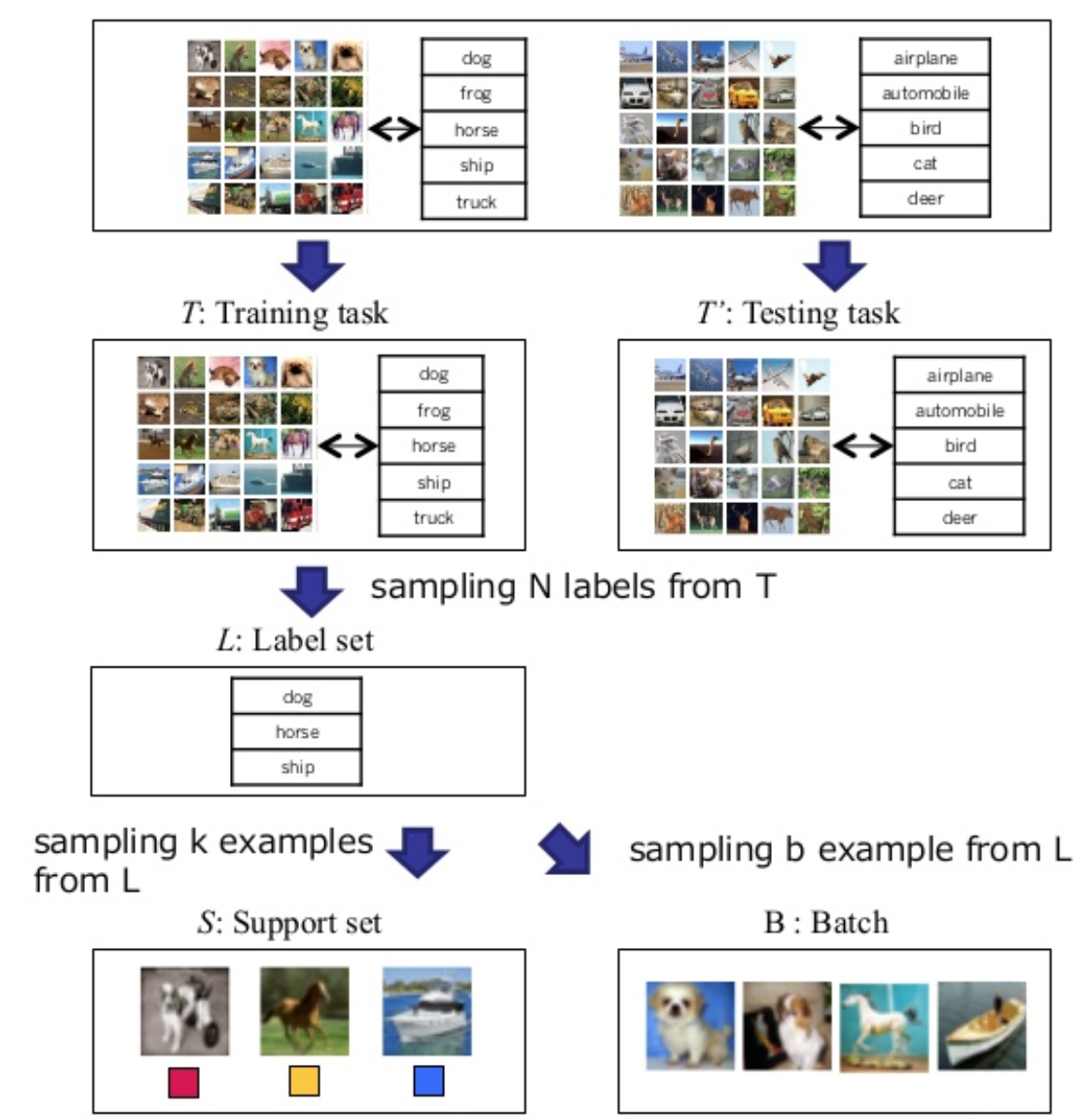For a similarity metric, two embeding functions $$f$$ and $$g$$ need to take similarity on feature space X. function $$g$$ has embeded $$x_{i}$$ independently from other elements but $$S$$ could be able to effect how be embeded $$\bar{x}$$ through function $$f$$. Then an attention kernel calculate cosin distance on these functions (similar to nearest neighbor).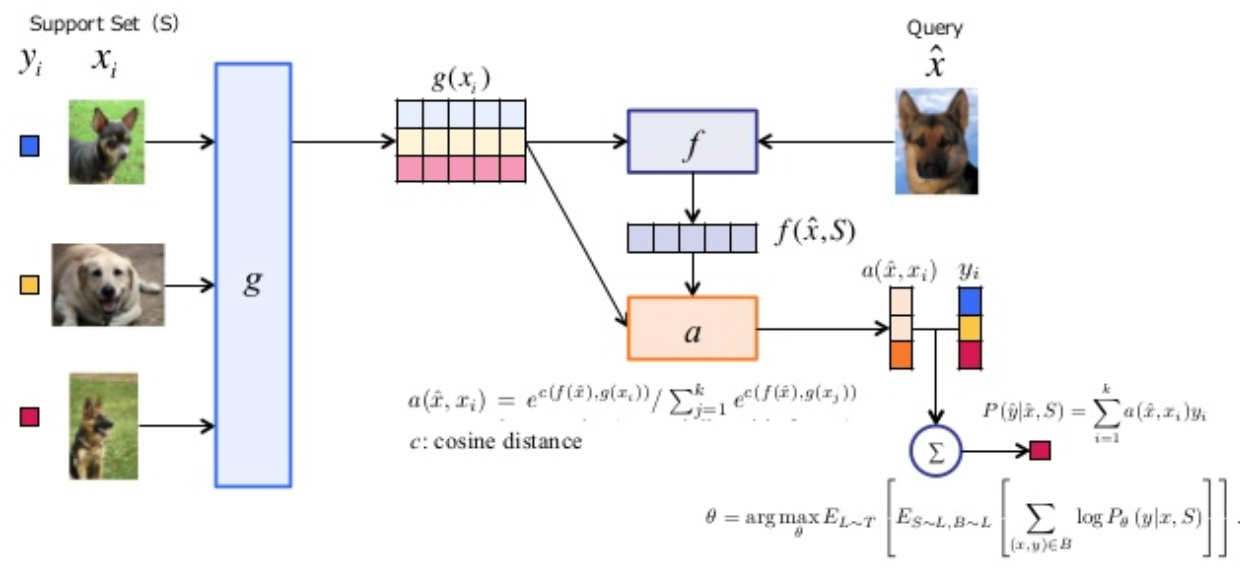The embeding of $$x_{i}$$ is a neural network (VGG) follow by a Bi-LSTM. The function f is a neural network (VGG) and then the embeding function g applied to each element $$x_{i}$$ to process the kernel for each set of S. Note that $$\bar{y}$$ is a linear combination of the lables in support set.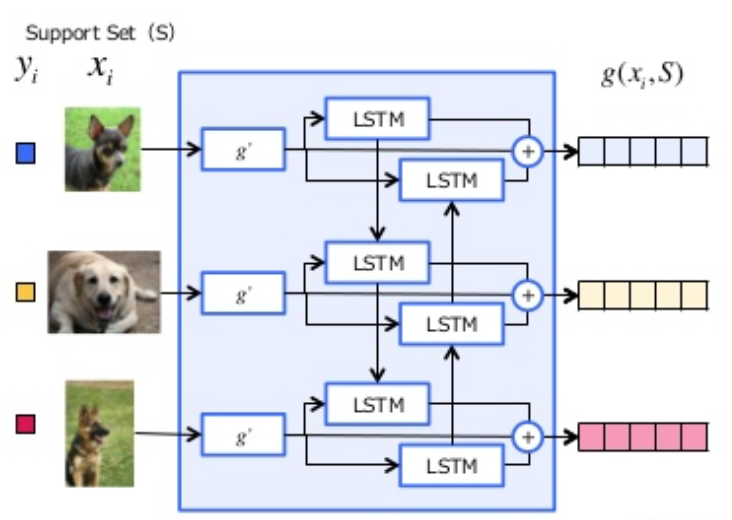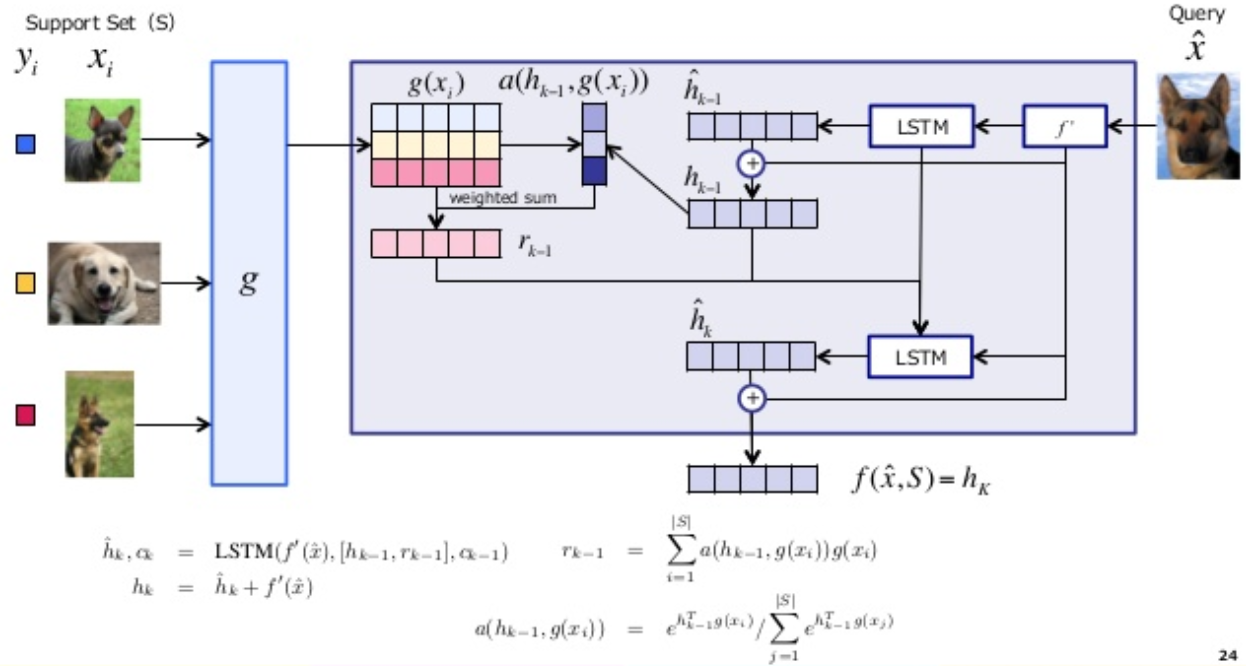### Results